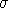## CGAL::Weighted_Minkowski_distance<Traits>

### Definition

The class Weighted_Minkowski_distance<Traits> provides an implementation of the concept OrthogonalDistance, with a weighted Minkowski metric on d-dimensional points defined by lp(w)(r,q)= (i=1i=d wi(ri-qi)p)1/p for 0 < p <and defined by l(w)(r,q)=max {wi |ri-qi|1id}. For the purpose of the distance computations it is more efficient to compute the transformed distancei=1i=d wi(ri-qi)p instead of the actual distance.

#include <CGAL/Weighted_Minkowski_distance.h>

### Parameters

Expects for the template argument a model of the concept SearchTraits, for example CGAL::Search_traits_2<Kernel>.

### Types

 Traits::FT FT; Number type. Traits::Point_d Point_d; Point type.

### Creation

Weighted_Minkowski_distance<Traits> wd ( int d);
Constructor implementing l2 metric for d-dimensional points.

template <class InputIterator>
 Weighted_Minkowski_distance wd ( FT power, int dim, InputIterator wb, InputIterator we);
Constructor implementing the lpower(weights) metric. power0 denotes the l(weights) metric. The values in the iterator range [wb,we) are the weight.

### Operations

FT wd.transformed_distance ( Point_d q, Point_d r)
Returns dpower, where d denotes the distance between q and r.

FT
 wd.min_distance_to_rectangle ( Point_d q, Kd_tree_rectangle r;)
Returns dpower, where d denotes the distance between the query item q and the point on the boundary of r closest to q.

FT
 wd.max_distance_to_rectangle ( Point_d q, Kd_tree_rectangle r;)
Returns dpower, where d denotes the distance between the query item q and the point on the boundary of r farthest to q.

FT
 wd.new_distance ( FT dist, FT old_off, FT new_off, int cutting_dimension)
Updates dist incrementally and returns the updated distance.

FT wd.transformed_distance ( FT d)
Returns dp for 0 < p <. Returns d for p=.

FT wd.inverse_of_transformed_distance ( FT d)
Returns d1/p for 0 < p <. Returns d for p=.